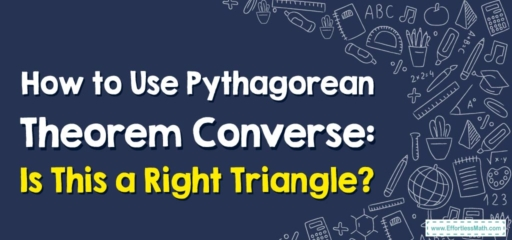# How to Use Pythagorean Theorem Converse: Is This a Right Triangle?

The Pythagorean Theorem is a fundamental concept in geometry that relates to the sides of a right triangle. It states that the square of the hypotenuse is equal to the sum of the squares of the other two sides. While the Pythagorean Theorem is widely known and used to find missing side lengths in right triangles, its converse is equally important in determining whether a given triangle is right. In this article, we will delve into the Pythagorean Theorem Converse and explore how it can be utilized to identify right triangles.## A Step-by-step Guide to Using Pythagorean Theorem Converse: Is This a Right Triangle?

Here’s how you can use the Pythagorean theorem converse to determine if a triangle is a right triangle:

### Step 1: Identify the Sides of the Triangle:

Determine which side of the triangle is the longest. This side is often referred to as the hypotenuse when you’re dealing with right triangles. Let’s label the sides of the triangle as follows: the longest side is ‘$$c$$’, and the other two sides are ‘$$a$$’ and ‘$$b$$’.

### Step 2: Square the Lengths of the Sides:

Square the lengths of all three sides. That is, calculate $$a^2, b^2$$, and $$c^2$$.

### Step 3: Check the Pythagorean Equation:

According to the Pythagorean theorem converse, if the triangle is a right triangle, then $$a^2 + b^2$$ should be equal to $$c^2$$.

If $$a^2 + b^2 = c^2$$, then the triangle is a right triangle.

If $$a^2 + b^2 > c^2$$, then the triangle is an acute triangle (all angles are less than $$90$$ degrees).

If $$a^2 + b^2 < c^2$$, then the triangle is an obtuse triangle (one angle is greater than $$90$$ degrees).

And that’s it! By following these steps, you can determine whether a given triangle is a right triangle using the converse of the Pythagorean theorem. Remember that the Pythagorean theorem and its converse only apply to triangles, not other polygons or shapes.

### What people say about "How to Use Pythagorean Theorem Converse: Is This a Right Triangle? - Effortless Math: We Help Students Learn to LOVE Mathematics"?

No one replied yet.

X
30% OFF

Limited time only!

Save Over 30%

SAVE $5 It was$16.99 now it is \$11.99